Online Tution   »   Sample Papers   »   Class 12 Chemistry Sample Paper 2023

# CBSE Class 12 Chemistry Sample Question Paper 2023

## Class 12 Chemistry Sample Paper 2023

CBSE (Central Board Of Secondary Education)  has introduced Sample Papers for students of classes 10 and 12 Board. These Sample papers are on its official website. Students can also download the sample papers from here given below.
CBSE has introduced sample papers for all the subjects according to the layout of the question paper which CBSE is going to implement in this session 2022-23. This time CBSE has introduced only one term examination which is going to be conducted at the end of the year in March- February 2023.
In this article, we have covered the CBSE Class 12 Chemistry Sample Paper. The students must solve CBSE Class 12 Chemisry Sample Paper which is given on this page and bookmark this page to get all the latest updates from CBSE regarding CBSE Annual Exam.

For scoring well in class 12th Click here

## Chemistry Class 12 Sample Question Paper 2023

SAMPLE PAPER (2022-23)

CHEMISTRY THEORY (043)

MM:70                                                                                                                                                                                                    Time: 3 hours

General Instructions:

Read the following instructions carefully.

1. There are 35 questions in this question paper with internal
2. SECTION A consists of 18 multiple-choice questions carrying 1 mark
3. SECTION B consists of 7 very short answer questions carrying 2 marks each.
4. SECTION C consists of 5 short answer questions carrying 3 marks
5. SECTION D consists of 2 case- based questions carrying 4 marks
6. SECTION E consists of 3 long answer questions carrying 5 marks
7. All questions are compulsory.
8. Use of log tables and calculators is not allowed

## Class 12 Chemistry Sample Paper 2023- SECTION A

The following questions are multiple-choice questions with one correct answer. Each question carries 1 mark. There is no internal choice in this section.

1. The major product of acid catalysed dehydration of 1-methylcyclohexanol is:
1. 1-methylcyclohexane
2. 1-methylcyclohexene
3. 1-cyclohexylmethanol
4. 1-methylenecyclohexane
2. Which one of the following compounds is more reactive towards SN1 reaction?
1. CH2=CHCH2Br
2. C6H5CH2Br
3. C6H5CH (C6H5)Br
4. C6H5CH(CH3) Br
3. KMnO4 is coloured due to:
1. d-d transitions
2. charge transfer from ligand to metal
3. unpaired electrons in d orbital of Mn
4. charge transfer from metal to ligand
4. Which radioactive isotope would have the longer half- life 15O or 19O? (Given rate constants for 15O and 19O are 5.63x 10-3 s-1 and k = 38 x 10-2s-1 respectively.)
1. 15O
2. 19O
3. Both will have the same half-life
4. None of the above, information given is insufficient
5. The molar conductivity of CH3COOH at infinite dilution is 390 Scm2/mol. Using the graph and given information, the molar conductivity of CH3COOK will be: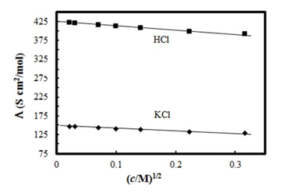1. 100 Scm2/mol
2. 115 Scm2/mol
3. 150 Scm2/mol
4. 125 Scm2/mol

*FOR VISUALLY CHALLENGED LEARNERS

*5. What is the molar conductance at infinite dilution for sodium chloride if the molar conductance at infinite dilution of Na+ and Cl- ions are 51.12 × 10-4 Scm2/mol and 73.54× 10-4 Scm2/mol respectively?

1. 66 Scm2/mol
2. 42 Scm2/mol
3. 20Scm2/mol
4. 78 Scm2/mol

6. For the reaction, A +2B àAB2, the order r.t. reactant A is 2 and w.r.t. reactant B. What will be change in rate of reaction if the concentration of A is doubled and B is halved?

1. increases four times
2. decreases four times
3. increases two times
4. no change

7. Arrange the following in the increasing order of their boiling points:

A : Butanamine, B: N,N-Dimethylethanamine, C: N- Etthylethanaminamine

1. C<B<A
2. A<B<C
3. A<C<B
4. B<C<A

8. TheCFSE of [CoCl6]3- is 18000 cm-1 the CFSE for [CoCl4] will be:

1. 18000 cm-1
2. 8000cm-1
3. 2000 cm-1
4. 16000 cm-1

9. What would be the major product of the following reaction?

C6H5 -CH2-OC6H5 + HBr à A + B

1. A= C6H5CH2OH , B= C6H6
2. A=C6H5CH2OH ,B= C6H5Br
3. A=C6H5CH3 ,B= C6H5Br
4. A=C6H5CH2Br , B= C6H5OH

10. Which of the following statements is not correct for amines?

1. Most alkyl amines are more basic than ammonia
2. pKb value of ethylamine is lower than
3. CH3NH2 on reaction with nitrous acid releases NO2
4. Hinsberg’s reagent reacts with secondary amines to form sulphonamides.

11. Which of the following tests/ reactions is given by aldehydes as well as ketones?

1. Fehling’s test
2. Tollen’s test
3. 2,4 DNP test
4. Cannizzaro reaction

12. Arrhenius equation can be represented graphically as follows: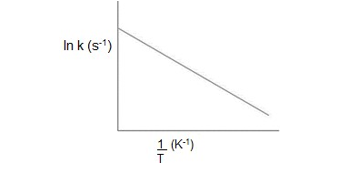The (i) intercept and (ii) slope of the graph are:

1. (i) ln A (ii) Ea/R
2. (i) A (ii) Ea
3. (i)ln A (ii) – Ea/R
4. (i) A (ii) -Ea

*FOR VISUALLY CHALLENGED LEARNERS

*12. The unit of rate constant for the reaction 2A + 2B → A2B2
which has rate = k [A]2[B] is:
a. mol L-1s-1
b. s-1
c. mol L-1
d. mol-2 L2 s-1

13. The number of ions formed on dissolving one molecule of FeSO4.(NH4)2SO4.6H2O in water is:

a. 3
b. 4
c. 5
d. 6

14. The oxidation of toluene to benzaldehyde by chromyl chloride is called
a. Etard reaction
b. Riemer-Tiemann reaction
c. Stephen’s reaction
d. Cannizzaro’s reaction

15. Given below are two statements labelled as Assertion (A) and Reason (R)

Assertion (A): An ether is more volatile than an alcohol of comparable molecular mass.
Reason (R): Ethers are polar in nature.
Select the most appropriate answer from the options given below:
a. Both A and R are true and R is the correct explanation of A
b. Both A and R are true but R is not the correct explanation of A.
c. A is true but R is false.
d. A is false but R is true.

16. Given below are two statements labelled as Assertion (A) and Reason (R)

Assertion (A): Proteins are found to have two different types of secondary structures viz alpha-helix and beta-pleated sheet structure.
Reason (R): The secondary structure of proteins is stabilized by hydrogen bonding.
Select the most appropriate answer from the options given below:
a. Both A and R are true and R is the correct explanation of A
b. Both A and R are true but R is not the correct explanation of A.
c. A is true but R is false.
d. A is false but R is true.

17. Given below are two statements labelled as Assertion (A) and Reason (R)

Assertion : Magnetic moment values of actinides are lesser than the theoretically predicted values.
Reason : Actinide elements are strongly paramagnetic.
Select the most appropriate answer from the options given below:
a. Both A and R are true and R is the correct explanation of A
b. Both A and R are true but R is not the correct explanation of A.
c. A is true but R is false.
d. A is false but R is true.

18. Given below are two statements labelled as Assertion (A) and Reason (R)

Assertion (A): Tertiary amines are more basic than corresponding secondary and primary amines in gaseous state.
Reason (R): Tertiary amines have three alkyl groups which cause +I effect.
Select the most appropriate answer from the options given below:
a. Both A and R are true and R is the correct explanation of A
b. Both A and R are true but R is not the correct explanation of A.
c. A is true but R is false.
d. A is false but R is true.

## CBSE Class 12 Chemistry Sample Paper 2023- SECTION B

This section contains 7 questions with internal choice in two questions. The following questions are very short answer type and carry 2 marks each.

19. A first-order reaction takes 69.3 min for 50% completion. What is the time needed for 80% of the reaction to get completed?
(Given: log 5 =0.6990, log 8 = 0.9030, log 2 = 0.3010)

20. Account for the following:
a. There are 5 OH groups in glucose
b. Glucose is a reducing sugar

OR

What happens when D – glucose is treated with the following reagents
a. Bromine water
b. HNO3

21. Give reason for the following:
a. During the electrophilic substitution reaction of haloarenes, para substituted derivative is the major product.
b. The product formed during SN1 reaction is a racemic mixture.
OR

a. Name the suitable alcohol and reagent, from which 2-Chloro-2-methyl propane can be prepared.
b. Out of the Chloromethane and Fluoromethane , which one is has higher dipole moment and why?

22. The formula Co(NH3)5CO3Cl could represent a carbonate or a chloride. Write the structures and names of possible isomers.

23. Corrosion is an electrochemical phenomenon. The oxygen in moist air reacts as follows:

O2(g) + 2H2O(l) + 4e– → 4OH– (aq).
Write down the possible reactions for corrosion of zinc occurring at anode, cathode, and overall reaction to form a white layer of zinc hydroxide.

24. Explain how and why will the rate of reaction for a given reaction be affected when
a. a catalyst is added
b. the temperature at which the reaction was taking place is decreased

25. Write the reaction and IUPAC name of the product formed when 2-Methylpropanal (isobutyraldehyde) is treated with ethyl magnesium bromide followed by hydrolysis.

## Class 12 Chemistry Sample Paper 2023- SECTION C

This section contains 5 questions with internal choice in two questions. The following questions are short answer type and carry 3 marks each.

26. Write the equations for the following reaction:
a. Salicylic acid is treated with acetic anhydride in the presence of conc.
H2SO4
b. Tert butyl chloride is treated with sodium ethoxide.
c. Phenol is treated with chloroform in the presence of NaOH

27. Using Valence bond theory, explain the following in relation to the paramagnetic complex [Mn(CN)6]3-
a. type of hybridization
b. magnetic moment value
c. type of complex – inner, outer orbital complex

28. Answer the following questions:
a. State Henry’s law and explain why are the tanks used by scuba divers filled with air diluted with helium (11.7% helium, 56.2% nitrogen and 32.1% oxygen)?
b. Assume that argon exerts a partial pressure of 6 bar. Calculate the solubility of argon gas in water. (Given Henry’s law constant for argon dissolved in water, KH = 40kbar)

29. Give reasons for any 3 of the following observations:
a. Aniline is acetylated before nitration reaction.
b. pKb of aniline is lower than the m-nitroaniline.
c. Primary amine on treatment with benzenesulphonyl chloride forms a product which is soluble in NaOH however secondary amine gives product which is insoluble in NaOH.
d. Aniline does not react with methyl chloride in the presence of anhydrous AlCl3 catalyst.

30. a. Identify the major product formed when 2-cyclohexylchloroethane undergoes a dehydrohalogenation reaction. Name the reagent which is used to carry out the reaction.
b. Why are haloalkanes more reactive towards nucleophilic substitution reactions than haloarenes and vinylic halides?

OR

a. Name the possible alkenes which will yield 1-chloro-1-methylcyclohexane on their reaction with HCl. Write the reactions involved.
b. Allyl chloride is hydrolysed more readily than n-propyl chloride. Why?

## Class 12 Chemistry Sample Paper 2023- SECTION D

The following questions are case-based questions. Each question has an internal choice and carries 4 (1+1+2) marks each. Read the passage carefully and answer the questions that follow.

31. Strengthening the Foundation: Chargaff Formulates His “Rules”
Many people believe that James Watson and Francis Crick discovered DNA in the 1950s. In reality, this is not the case. Rather, DNA was first identified in the late 1860s by Swiss chemist Friedrich Miescher. Then, in the decades following Miescher’s discovery, other scientists–notably, Phoebus Levene and Erwin Chargaff–carried out a series of research efforts that revealed additional details about the DNA molecule, including its primary chemical components and the ways in which they joined with one another. Without the scientific foundation provided by these pioneers, Watson and Crick may never have reached their groundbreaking conclusion of 1953: that the DNA molecule exists in the form of a three-dimensional double helix.
Chargaff, an Austrian biochemist, as his first step in this DNA research, set out to see whether there were any differences in DNA among different species. After developing a new paper chromatography method for separating and identifying small amounts of organic material, Chargaff reached two major conclusions:
(i) the nucleotide composition of DNA varies among species.
(ii) Almost all DNA, no matter what organism or tissue type it comes from maintains certain properties, even as its composition varies. In particular, the amount of adenine (A) is similar to the amount of thymine (T), and the amount of guanine (G) approximates the amount of cytosine (C). In other words, the total amount of purines (A + G) and the total amount of pyrimidines (C + T) are usually nearly equal. This conclusion is now known as “Chargaff’s rule.”
Chargaff’s rule is not obeyed in some viruses. These either have single- stranded DNA or RNA as their genetic material.
Answer the following questions:

a. A segment of DNA has 100 adenine and 150 cytosine bases. What is the total number of nucleotides present in this segment of DNA?
b. A sample of hair and blood was found at two sites. Scientists claim that the samples belong to same species. How did the scientists arrive at this conclusion?
c. The sample of a virus was tested and it was found to contain 20% adenine, 20% thymine, 20 % guanine and the rest cytosine. Is the genetic material of this virus (a) DNA- double helix (b) DNA-single helix (c) RNA? What do you infer from this data?

OR

How can Chargaff’s rule be used to infer that the genetic material of an organism is double- helix or single- helix?

32. Henna is investigating the melting point of different salt solutions.
She makes a salt solution using 10 mL of water with a known mass of NaCl salt. She puts the salt solution into a freezer and leaves it to freeze.
She takes the frozen salt solution out of the freezer and measures the temperature when the frozen salt solution melts.
She repeats each experiment.

 S.No Mass of the salt used in g Melting point in 0C Readings Set 1 Reading Set 2 1 0.3 -1.9 -1.9 2 0.4 -2.5 -2.6 3 0.5 -3.0 -5.5 4 0.6 -3.8 -3.8 5 0.8 -5.1 -5.0 6 1.0 -6.4 -6.3

Assuming the melting point of pure water as 0oC, answer the following questions:
a. One temperature in the second set of results does not fit the pattern. Which temperature is that? Justify your answer.
b. Why did Henna collect two sets of results?
c. In place of NaCl, if Henna had used glucose, what would have been the melting point of the solution with 0.6 g glucose in it?

OR
What is the predicted melting point if 1.2 g of salt is added to 10 mL of water? Justify your answer.

## Class 12 Chemistry Sample Paper 2023- SECTION E

The following questions are long answer type and carry 5 marks each. Two questions have an internal choice.

33. a. Why does the cell voltage of a mercury cell remain constant during its

b. Write the reaction occurring at anode and cathode and the products of electrolysis of aq KCl.
c. What is the pH of HCl solution when the hydrogen gas electrode shows a potential of -0.59 V at standard temperature and pressure?

OR

a. Molar conductivity of substance “A” is 5.9×103 S/m and “B” is 1 x 10-16 S/m. Which of the two is most likely to be copper metal and why?
b. What is the quantity of electricity in Coulombs required to produce 4.8 g of Mg from molten MgCl2? How much Ca will be produced if the same amount of electricity was passed through molten CaCl2? (Atomic mass of Mg = 24 u, atomic mass of Ca = 40 u).
c. What is the standard free energy change for the following reaction at room temperature? Is the reaction spontaneous?
Sn(s) + 2Cu2+ (aq) à Sn2+ (aq) + 2Cu+ (s)
34.A A hydrocarbon (A) with molecular formula C5H10 on ozonolysis gives two products
(B) and ( C). Both (B) and (C) give a yellow precipitate when heated with iodine in presence of NaOH while only (B) give a silver mirror on reaction with Tollen’s reagent.
a. Identify (A), (B) and (C).
b. Write the reaction of B with Tollen’s reagent
c. Write the equation for iodoform test for C
d. Write down the equation for aldol condensation reaction of B and C.

OR

An organic compound (A) with molecular formula C2Cl3O2H is obtained when (B) reacts with Red P and Cl2. The organic compound (B) can be obtained on the reaction of methyl magnesium chloride with dry ice followed by acid hydrolysis.
a. Identify A and B
b. Write down the reaction for the formation of A from B. What is this reaction called?
c. Give any one method by which organic compound B can be prepared from its corresponding acid chloride.
d. Which will be the more acidic compound (A) or (B)? Why?
e. Write down the reaction to prepare methane from the compound (B).
35. Answer the following:
a. Why are all copper halides known except that copper iodide?
b. Why is the Eo(V3+/V2+) value for vanadium comparatively low?
c. Why HCl should not be used for potassium permanganate titrations?

## CBSE Class 12 Chemistry Sample Paper 2023 PDF Download

Chemistry-Sample Question Paper PDF

## Class 12 Chemistry Sample Paper 2023 Marking Scheme

Chemistry-Marking Scheme PDF

## Class 12 Chemistry Sample Paper 2023- FAQs

Q. Do the exam evaluators give marks based on a good presentation on the answer sheet?
Ans: No, there is no provision to give marks for a good presentation. However, students should concentrate on writing well-structured, neat, and highlighted answers. Students can also see the CBSE toppers answer sheet to learn how to write an answer.

Q. Is it important to write down all I learn as I study for the CBSE Class 12 board exam?
Ans: No, it is not necessary to write everything down, making notes of the most important information will help you to understand the chapter and boost your confidence.

Q.Will questions directly ask from the CBSE Class 12 board sample papers?
Ans: You can better understand the format, structure, and question kinds using CBSE 12th sample papers. Any topic in the syllabus may be included in exam questions.

Sharing is caring!

Thank You, Your details have been submitted we will get back to you.
•CBSE Sample Paper 2022- 23 Class 12 for ...
•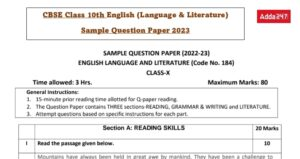Class 10 English Sample Paper 2022- 23 w...
•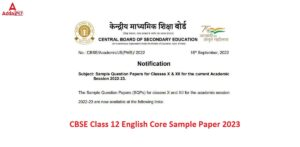Class 12 English Core Sample Paper 2022-...
•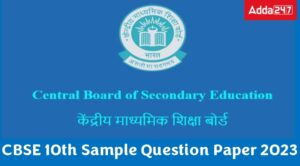CBSE Sample Paper 2022-23 For Class 10
•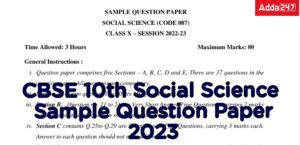Class 10 Social Science (SST) Sample Pap...
•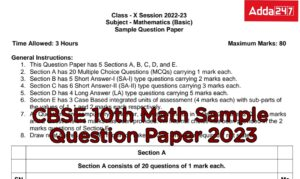CBSE Class 10 Maths Sample Paper 2022- 2...